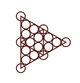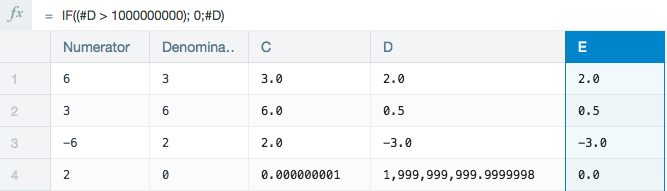• Official commentJoel Stewart

<error> statuses cannot be converted to another value. Can you share the details of the <error> value that you are observing so that we can help to prevent this error from occurring in the first place.

•Jacob Cluff

I'm also interested in a solution to this.

•ArunKumar

thanks a lot.We share the awesome posts.I will be learning about that lot of new information.

http://www.informaticatrainingchennai.in

•James Watkins

Hey Joel, I am having a similar issue. Long story short, I have an issue where my numerator is 0 and my denominator is >0. Naturally i am getting a divide by 0 error. how do i go about getting this error to just be 0?

•Alan Mark

Hi James,

To know if we can do something about this we'll need to know how you're ending up with a *denominator* of 0 (numerator is divided by denominator).

Traditionally if you're dividing one column by another, I would do an operation on the denominator column that changes all 0 values into a very, very very small value.  This will lead to an impossibly large number that makes no sense for the dataset.  This will get around the divide by zero and you won't see <error>, just a huge number.  Then you can use an IF statement in a new column to convert numbers over a certain amount into whatever you want.

Say I have two columns, Numerator and Denominator.  In this example we'll say numerator is 2 and denominator is 0.

First you will do IF((#Denominator == 0); 0.0000000001; #Denominator)

This will transform the 0 into a very tiny number in a new column, we'll just refer to as C.

Now on a new column D, do Div(#Numerator;#C)

Now we have a D column with a giant number, then you do IF((#D > 1000000000); 0; #D)

Now that result will be 0.

PFA attached screenshot.•Jay Lalchandani

Hi James,

You can use an IF function to isolate the zero denominator values.

Based on Alan's workbook above :

IF(#Denominator == 0; 0 ; Div(#Numerator;#Denominator))

This function states if the Denominator is equal to 0, then the resulting value should be 0.  In all other cases, Numerator is divided by denominator.